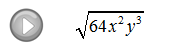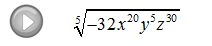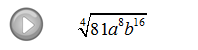## Pages

Now when working with square roots and variables we should be a bit careful.

The variable could represent a positive or negative number so we must ensure that it is positive by making use of the absolute value.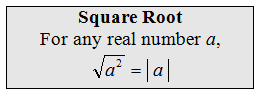To avoid this technicality many textbooks state, at this point, that we assume all variables are positive. If not, use the absolute values as in the following problems.
Simplify. (Assume variables could be negative.)To avoid many of the technicalities when working with nth roots we will assume, from this point on, that all the variables are positive.

Simplify. (Assume all variables represent positive numbers.)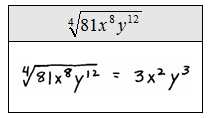Students often try to simplify the previous problem. Be sure to understand what makes the following problems all different.The property: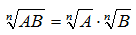says that we can simplify when the operation is multiplication.  There is no corresponding property for addition or subtraction.